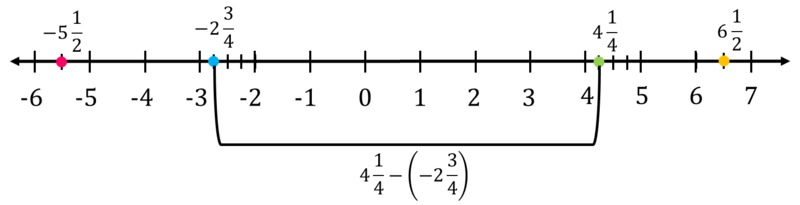# Distances Between Houses

Alignments to Content Standards: 7.NS.A.1

Aakash, Bao Ying, Chris and Donna all live on the same street as their school, which runs from east to west.

• Aakash lives $5 \frac{1}{2}$ blocks to the west.
• Bao Ying lives $4 \frac{1}{4}$ blocks to the east.
• Chris lives $2 \frac{3}{4}$ blocks to the west.
• Donna lives $6 \frac{1}{2}$ blocks to the east.
1. Draw a picture that represents the positions of their houses along the street.
2. Find how far is each house from every other house?
3. Represent the relative position of the houses on a number line, with the school at zero, points to the west represented by negative numbers, and points to the east represented by positive numbers.
4. How can you see the answers to part (b) on the number line? Using the numbers (some of which are positive and some negative) that label the positions of houses on the number line, represent these distances using sums or differences.

## IM Commentary

The purpose of this task is for students to solve a problem involving distances between objects whose positions are defined relative to a specified location and to see how this kind of situation can be represented with signed numbers.

A full solution requires systematic listing of pairs of houses, a valid list of differences - either subtracting the smaller number from the larger, or taking absolute value of difference in any order - as well as computations which could be done by counting on the number line or subtracting fractions. Note as well the "twist" with the houses not in "alphabetical" order on the number line requires students to make sense of the problem (MP 1)attend to precision (MP 6).

This problem framework could be used with integer values (say to enhance understanding of 6.NS.5) or later for tasks involving the Pythagorean Theorem in the plane (8.G.8).

## Solution

1. There are many ways to draw a picture that represents this situation. Here is one:2. Here is a table that shows the distances between each of the student's houses.
Bao Ying Chris Donna $9\frac34$ $2\frac34$ $12$ $7$ $2\frac14$ $9\frac14$
3. The colors show which point corresponds to which person in the first picture:4. The distance between the houses is represented by the distance between the points that correspond to the houses on the number line. This can be computed by subtracting the numbers that represent the position of the house relative to the school. For example, to find the distance between Bao Ying and Chris, we subtract $-2\frac34$ from $4\frac14$: $$4\frac14-(-2\frac34)$$ We can communicate this more clearly by labeling the distance between the points with the difference of the numbers on the number line: